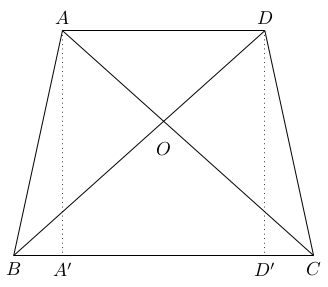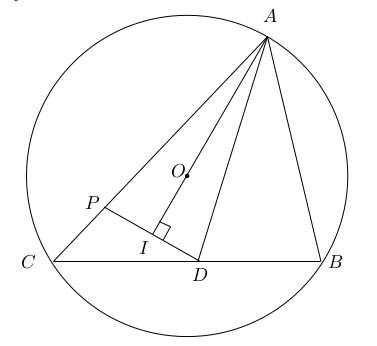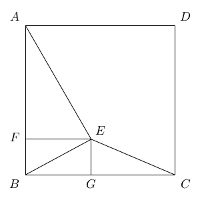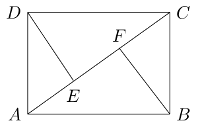Year:The 2017 Canadian Open Mathematics Challenge — Nov 2/3Nicolae Strungaru

## Problem of the Week

by Assoc. Prof. Nicolae Strungaru, Grant MacEwan University

The COMC has three parts. In part A solutions do not require work be shown and may be possible to do in your head. In part B the problems begin to draw on more knowledge and have some more challenging aspects that will need a pencil and paper to solve. By Part C the problems require that work be shown and involve arguments to support the answer.

We have selected a cross section of contest problems from a variety of national and regional contests that we hope will stimulate interest in problem solving and give some experience to get ready to write the Canadian Open Mathematics Competition in November. The problem areas are not tied to particular grade levels, or to the curriculum but cover a number of areas from algebra, through logic and some geometry.

We will post solutions to these problems one week later, but teachers should be aware that determined students may be able to locate solutions elsewhere online before then.

For a more comprehensive set of problems and solutions at each of these levels, please feel welcome to download past official exams and solutions from our archive.

Need more COMC Problems-of-the-Week? Take a look at the set from past years: 2016, 2015, 2014, or 2013!

Week 9

• Problem (posted October 31st)

The area of a trapezoid is 2 cm$^2$ and the sum of its diagonal is $4$ cm. Find the altitude of the trapezoid.

• Solution (posted November 7th)

This is problem 4 of the Georgian Math Olympiad 1997, Grade X, which appeared in Crux Mathematicorum in [2000:193]. We give the solution of Amengual Covas that appeared in Crux Mathematicorum in [2002:204-205], modified by the editor.

Let $AD$ be the small base of $ABCD$ and $CD$ the large base.We first prove that the diagonals are equal and perpendicular at $O$.

We have \begin{align} \mbox{Area} (ABD) &= \frac{1}{2} BD \cdot AO \cdot \sin(\angle AOB) \cr \mbox{Area} (CBD) &= \frac{1}{2} BD \cdot CO \cdot \sin(\angle COD) \cr 2=\mbox{Area} (ABCD) &= \frac{1}{2} BD \cdot AC \cdot \sin(\angle AOB) \cr \end{align}

Let us denote $BD=x$. Then $AC=4-x$, and hence $$4= x (4-x) \sin(\angle AOB) \leq x(4-x) =4x-x^2=4-(x-2)^2 \leq 4 \,.$$

Therefore, all inequalities in the above line are equalities. This implies that \begin{align} 4-(x-2)^2 &= 4 \qquad \mbox{and} \cr x (4-x) \sin(\angle AOB) &= x(4-x). \cr \end{align}

The first equality gives $x=2$, and therefore $BD=x=2=4-x=AC$. The second equality gives $\angle AOB=90^\circ$ and hence $AC \perp BD$.

Next, we show that $AB=CD$, that is the trapezoid is isosceles. To see this, drop perpendiculars $AA',DD'$ from $A$ and $D$, respectively, on the side $BC$.

We have \begin{align} AC &=BD \cr AA' &= DD' \cr \angle AA'C & =\angle DD'B= 90^\circ \cr \end{align}

This implies that the right triangles $AA'C$ and $DD'B$ are congruent, and hence $A'C=BD'$. From here, we get $$A'B=CD' \,.$$

Now, the right triangles $AA'B$ and $DD'C$ are congruent, since \begin{align} A'B &= D'C \cr AA' &= DD' \cr \angle AA'B & =\angle DD'C= 90^\circ \cr \end{align} and hence $AB=CD$.

Since $ABCD$ is an isosceles trapezoid, it follows immediately that $BOC$ is an isosceles right triangle, and hence $\angle OCB=45^\circ$. From here, we get that $AA'=A'C$.

Finally \begin{align} 2 &= \mbox{area}(ABCD) = \mbox{area}(ABA')+ \mbox{area}(AA'D'D)+ \mbox{area}(CDD') \cr &= \mbox{area}(AA'D'D)+ 2\mbox{area}(CDD') \cr &= AA' \cdot A'D'+ DD' \cdot D'C= AA'\cdot A'C \cr \end{align}

Since $AA'=A'C$ we get that $A'A^2=2$ and hence $$AA'=\sqrt{2} \,.$$

Week 8

• Problem (posted October 24th)

An acute triangle $ABC$ is inscribed in a circle with the center at $O$. The bisector of $\angle A$ meets the side $BC$ at $D$. The perpendicular from $D$ to $AO$ meets the side $AC$ at a point $P$ which is interior to $AC$. Show that $AB=AP$.

• Solution (posted October 31st)

This is problem 5 of the 1995 Italian Math Olympiad which appeared in Crux Mathematicorum in [1998:323-324]. We give the solution of Pierre Bornsztein that appeared in Crux Mathematicorum in [2000:79-80].Since $ABC$ is acute, $O$ is interior to $ABC$. Since $P$ is interior to $AC$, $O$ is interior to $ADC$.

Let us denote as usual $\angle A= \alpha, \angle B= \beta$ and $\angle C= \gamma$. We have \begin{align} \angle AOC &= 2 \beta \cr AO&=OC \cr \end{align} and therefore, $$\angle CAO = \frac{1}{2} ( 180^\circ -2 \beta) =90^\circ - \beta \,.$$

This yields $$\angle OAD = \frac{\alpha}{2}-\left(90^\circ - \beta \right)=\frac{\alpha}{2} +\beta -90^\circ \,.$$

Let us denote by $I$ the intersection between $AO$ and $PD$. Then, the triangle $\Delta AID$ is right at $I$ and hence \begin{align} \angle PDA &=\angle IDA = 90^\circ - \angle OAD \cr &=180^\circ - \frac{\alpha}{2}-\beta \cr &=180^\circ - \angle DAB -\angle DBA =\angle ADB \,.\cr \end{align}

This shows that the triangles $\Delta PDA$ and $\Delta BDA$ are congruent, and hence $PA=BA$.

Week 7

• Problem (posted October 17th)

Do there exist a number $q \in \mathbb N$ and a prime number $p \in \mathbb N$ such that $$3^p+7^p = 2 \cdot 5^q?$$

• Solution (posted October 24th)

This is problem 4 of the Ukrainian Math Olympiad, 11th Grade, which appeared in Crux Mathematicorum in [2006:217-218]. We give the solution of Michel Bataille that appeared in Crux Mathematicorum in [2007:227-228].

There is no such pair $(p,q)$. To prove this, we argue by contradiction.

Assume by contradiction that a pair $(p,q)$ exists. It is straightforward to check that $p=2$ doesn't work. Then, $p$ is odd, and hence $$2 \cdot 5^q=3^p+7^p=10 \cdot (3^{p-1}-3^{p-2}\cdot 7+...-3 \cdot 5^{p-2}+7^{p-1}).$$

It follows that \begin{align} 3^{p-1}-3^{p-2}\cdot 7+...-3 \cdot 7^{p-2}+7^{p-1}&=5^{q-1} \,.\tag{1}\cr \end{align}

A fast computation shows that $q=1$ cannot satisfy the initial equation, and hence $5^{q-1} \equiv 0 \pmod{5}$. Now, using $3\equiv -2 \pmod{5}$ and $7\equiv 2 \pmod{5}$, and $p$ odd, in (1) we get $$2^{p-1}+2^{p-1}+...+2^{p-1}=p2^{p-1} \equiv 0 \pmod{5} \,.$$

This implies that $p=5$ and hence $$2 \cdot 5^2 \cdot 341=3^5+7^5 = 2 \cdot 5^q \,,$$ which is a contradiction.

Week 6

• Problem (posted October 10th)

This week we look at a problem about two polynomials.

Let $P(X)$ and $Q(X)$ be two polynomials with integer coefficients. If $\frac{P(n)}{Q(n)}$ is an integer for every integer $n$, prove that there exists a polynomial $S(X)$ with rational coefficients such that $P(X)=Q(X)S(X)$.

• Solution (posted October 17th)

This is problem 5 of Alberta High School Math Competition 1995, Part B. We present the official solution.

We divide $P(X)$ by $Q(X)$ and obtain a quotient $S(X)$ and a remainder $R(X)$. Then, we have $$P(X)=Q(X)S(X)+R(X) \,,$$ with $\deg(R(X)) < \deg(Q(X))$. We then have: \begin{align} \frac{P(X)}{Q(X)}&= S(X)+ \frac{R(X)}{Q(X)} \,.\tag{1}\cr \end{align}

Since both $P(X)$ and $Q(X)$ have integer coefficients, $S(X)$ has rational coefficients. To complete the proof we show that $R(X)=0$.

We can write $$S(X)=\frac{1}{m} T(X) \,,$$ for some integer $m > 0$ and some polynomial with integer coefficients. By combining the hypothesis with (1) we then get that for all integers $n$ we have $$m \frac{R(n)}{Q(n)} = m\frac{P(n)}{Q(n)}-T(n) \in \mathbb Z \,.$$

Since $\deg(Q(x)) > \deg(R(x))$, for $x$ sufficiently large we have $$\left| m \frac{R(x)}{Q(x)} \right| < 1\,.$$

Therefore, for all integers $n$ which are sufficiently large we get that $\left| m \frac{R(n)}{Q(n)} \right|=0$ and hence, that $R(n)=0$. This shows that $R$ has infinitely many roots, and thus $R=0$, as claimed.

Editor note: Note that we cannot conclude that $S(x)$ has integer coefficients. Indeed, if $P(X)=X^2+X$ and $Q(X)=2$ then $\frac{P(n)}{Q(n)}=\frac{n(n+1)}{2}$ is an integer for all integers $n$ but $S(X)=\frac{1}{2}X^2+\frac{1}{2}$.

Pólya proved that a polynomial $P(X)$ takes integer values at all integers if and only if there exists some $n$ and integers $a_0,..,a_n$ such that \begin{align} P(X)&= a_n \frac{X(X-1)...(X-n+1)}{n!}+a_{n-1} \frac{X(X-1)+...+(X-n+2)}{(n-1)!}+...\cr &+a_{2} \frac{X(X-1)}{2!}+a_1X+a_0 \,.\cr \end{align}

See if you can prove this!

Week 5

• Problem (posted October 3rd)

The sequence $a_n$ is defined by $a_1=1$ and for all $n \geq 1$ $$a_{n+1}=\frac{a_n}{1+n a_n} \,.$$

Find $a_{1996}$.

• Solution (posted October 10th)

This is problem 5 of the 1999 Íslenzka Staerŏfræŏikeppni Framhaldsskólanema which appeared in Crux Mathematicorum in [2001:232-233]. We give the solution of Michel Bataille that appeared in Crux Mathematicorum in [2003:304].

It is easy to see that $a_n >0$ for all $n$. Then, for all $n$ we have $$\frac{1}{a_{n+1}}=\frac{1}{a_n}+n \,.$$

Therefore, \begin{align} \frac{1}{a_{1996}}-1 &= \frac{1}{a_{1996}}-\frac{1}{a_1}= \sum_{k=1}^{1995} \left( \frac{1}{a_{k+1}}-\frac{1}{a_k} \right) \cr &= \sum_{k=1}^{1995} k =\frac{1995 \cdot 1996}{2} =1991010 \cr \end{align}

Therefore $$a_{1996}=\frac{1}{1991011} \,.$$

Week 4

• Problem (posted September 26th)

Find all polynomials $P(X)$ satisfying $$(X-16)P(2X)=16(X-1)P(X) \,.$$

• Solution (posted October 3rd)

This is problem 3 of the 10th Irish Math Olympiad 1997, which appeared in Crux Mathematicorum in [2001:6-8], with solution appearing in Crux Mathematicorum in [2003:96]. We give an alternate solution.

Setting $X=16$ in the given equation we get $P(16)=0$ and hence, we can write $P(X)=(X-16)P_1(X)$ for some polynomial $P_1(X)$. The given relation then becomes $$(X-8)P_1(2X)=8(X-1)P_1(X) \,.$$

Setting $X=8$ we get $P_1(8)=0$ and hence, we can write $P_1(X)=(X-8)P_2(X)$ for some polynomial $P_2(X)$. Thus, we get $$(X-4)P_2(2X)=4(X-1)P_2(X) \,.$$

Setting $X=4$ we get $P_2(4)=0$ and hence, we can write $P_2(X)=(X-4)P_3(X)$ for some polynomial $P_3(X)$. Thus, we get $$(X-2)P_3(2X)=2(X-1)P_3(X) \,.$$

Setting $X=2$ we get $P_3(2)=0$ and hence, we can write $P_3(X)=(X-2)P_4(X)$ for some polynomial $P_4(X)$. Thus, we get $$P_4(2X)=P_4(X) \,.$$

Then, we have $P_4(1)=P_4(2)=P_4(4)=P_4(8)=...=P_4(2^n)=..$ and hence $P_4(X)=a$ for some constant $a$.

Answer: $P(X)=a(X-16)(X-8)(X-4)(X-2)$.

Week 3

• Problem (posted September 19th)

This week we look at a point inside a square.

A point $E$ inside a square $ABCD$ is such that $AE=5, BE=2\sqrt{2}$ and $CE=3$. Determine the area of $ABCD$.

• Solution (posted September 26th)

This is problem 3 of Alberta High School Math Competition 2002, Part B. We present the solution of Keith Chung from the contest.

Drop perpendiculars $EF$ and $EG$ from $E$ onto $AB$ and $AC$ respectively.Denote \begin{align} EF & = x \cr EG & =y \cr AB & =s \,. \end{align}

Then \begin{align} x^2+y^2&=8 \tag{1}\cr (s-x)^2+y^2&=9\tag{2}\cr x^2+(s-y)^2&=25 \tag{3} \end{align}

From (1) and (2) we get $s^2-2sx=1$ and hence $$x=\frac{s^2-1}{2s} \,.$$

From (1) and (3) we get $s^2-2sy=17$ and hence $$x=\frac{s^2-17}{2s} \,.$$

Plugging back in (1) we get $$(s^2-1)^2+(s^2-17)^2=32s^2 \,,$$

or, equivalently $$(s^2-5)(s^2-29)=0 \,.$$

Since $E$ is inside $ABCD$ and $AE=5$, we cannot have $s=\sqrt{5}$. Therefore $s^2=29$, so the desired area is 29.

Week 2

• Problem (posted September 12th)

This week we look at an equation with integer roots.

Determine all rational numbers $r$ for which all the solutions of the equation $$rx^2+(r+1)x+r-1=0$$ are integers.

• Solution (posted September 19th)

Problem 5 of the $21^{\mbox{st}}$ Austian Mathematical Olympiad, Final Round, 1990, which appeared in Crux Mathematicorum [1992:100]. We present the similar solutions by Joseph Ling, Pavlos Maragoudakis and Michael Selby which appeared at [1993:138].

If $r=0$ then the equation becomes $x-1=0$, so $x=1$. Therefore $r=0$ is a solution.

If $r \neq 0$, let then $x_1 \leq x_2$ be the roots of the quadratic equation. Then \begin{align} x_1+x_2 &= -\frac{r+1}{r}=-1-\frac{1}{r} \cr x_1x_2 &= \frac{r-1}{r}=1-\frac{1}{r} \,. \end{align}

Then \begin{align} x_1x_2-(x_1+x_2) &= 2 \cr (x_1-1)(x_2-1) &= 3 \,. \end{align}

Therefore, as $x_1 \leq x_2$ are integers, we either have $x_1=2, x_2=4$ or $x_1=-2, x_2=0$.

In the first case we get $r=-\frac{1}{7}$ while in the second we get $r=1$.

Answer: $r \in \{ -\frac{1}{7}, 0, 1 \}$.

Week 1

• Problem (posted September 5th)

We give two entry level problems this week. Give them a try. Look for the source and the solution next week!

Problem A
Prove that the number $$\underbrace{111...111}_{1997} \underbrace{222...222}_{1998} 5$$ is a perfect square.

Problem B
In the diagram, $ABCD$ is a rectangle with $AD=1$, and both $BF$ and $DE$ are perpendicular to the diagonal $AC$. We further have $AE=EF=FC$. Find the length of the side $AB$.• Solution (posted September 12th)

Problem A

Problem 1 of the 2nd Junior Balkan Math Olympiad, 1998, which appeared in the Skoliad Corner of Crux Mathematicorum at [2002:522]. We present the official solution that appeared at [2003:262].

\begin{align} \underbrace{111...111}_{1997}\underbrace{222...222}_{1998}5 &=\underbrace{111...111}_{1997}\underbrace{000...000}_{1999}+2\cdot \underbrace{111...111}_{1998}0+5 \cr & = \frac{10^{1997}-1}{9}\cdot10^{1999}+2 \cdot \frac{10^{1998}-1}{9}\cdot10+5\cr & =\frac{1}{9} \left(10^{3996}-10^{1999}+2\cdot10^{1999}-20+45 \right) \cr & =\frac{1}{9} \left(10^{3996}+\cdot10^{1999}+25 \right) \cr & =\frac{1}{9} \left(10^{3996}+2 \cdot 5 \cdot10^{1998}+5^2 \right) \cr & =\frac{1}{9} \left(10^{1998}+5 \right)^2 =\left(\frac{10^{1998}+5}{3}\right)^2=\left(\frac{10^{1998}-1}{3}+2\right)^2 \cr &=\left( \underbrace{333...333}_{1998}+2 \right)^2 \end{align}

Problem B

Problem 10 of the British Columbia Secondary School Mathematics Contest, 2008, Junior Final, Part A which appeared in the Skoliad Corner of Crux Mathematicorum at [2008:321-324]. We present the solution by Jixuan Wang that appeared at [2009:269].

Let $x$ be the common length of $AE, EF$ and $FC$. Then, $EC=2x$.

By the Pythagorean Theorem, $AE^2+DE^2=AD^2$ and hence $$DE=\sqrt{1-x^2} \,.$$

Note that $$\angle ECD =90^\circ -\angle EDC =\angle ADE \,,$$ and therefore $$\Delta DEA \sim \Delta CED \,.$$

We therefore get \begin{align} \frac{EA}{DE} &=\frac{ED}{CE} \qquad \Rightarrow \cr \frac{x}{\sqrt{1-x^2}} &= \frac{\sqrt{1-x^2}}{2x} \qquad \Rightarrow \cr 2x^2&=1-x^2 \qquad \Rightarrow \cr x&=\frac{1}{\sqrt{3}} \end{align}

Thus, $AC=3x =\sqrt{3}$ and by the Pythagorean Theorem in $\Delta ABC$ we get $$AB= \sqrt{2} \,.$$